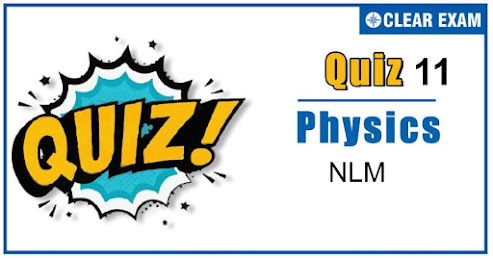## [LATEST]\$type=sticky\$show=home\$rm=0\$va=0\$count=4\$va=0

As per analysis for previous years, it has been observed that students preparing for NEET find Physics out of all the sections to be complex to handle and the majority of them are not able to comprehend the reason behind it. This problem arises especially because these aspirants appearing for the examination are more inclined to have a keen interest in Biology due to their medical background. Furthermore, sections such as Physics are dominantly based on theories, laws, numerical in comparison to a section of Biology which is more of fact-based, life sciences, and includes substantial explanations. By using the table given below, you easily and directly access to the topics and respective links of MCQs. Moreover, to make learning smooth and efficient, all the questions come with their supportive solutions to make utilization of time even more productive. Students will be covered for all their studies as the topics are available from basics to even the most advanced. .

Q1. A bird is sitting in a large closed cage which is placed on a spring balance. It records a weight of 25 N. The bird (mass m=0.5 kg) files upward in the cage with an acceleration of 2 m/s^2. The spring balance will now record a weight of
•  24 N
•  25 N
•  26 N
•  27 N
Solution
Reading =Weight of cage + Reaction by bird =20+0.5(10+2)=26 N .

Q2. A body weight 8 g when placed in one pan and 18 g when placed on the other pan of a false balance. If the beam is horizontal when both the pans are empty. The true weight of the body is
•  13 g
•  12 g
•  15.5 g
•  15 g
Solution

Q3. A block of mass 5 kg is on a rough horizontal surface and is at rest. Now a force of 24 N is imparted to it with negligible impulse. If the coefficient of kinetic friction is 0.4 and g=9.8 m/s^2, then the acceleration of the block is
•  0.26 m/s2
•  0.39 m/s2
•  0.69 m/s2
•  0.88 m/s2
Solution
Net force =Applied force - Friction force ma=24-μmg=24-0.4×5×9.8=24-19.6 ⇒a=4.4/5=0.88 m/s2
Q4. A cork is submerged in water by a spring attached to the bottom of a pail. When the pail is kept in a elevator moving with an acceleration downwards, the spring length
•  Increases
•  Decreases
•  Remains unchanged
•  Data insufficient
This causes elongation in the spring. When the lift moves down with acceleration a, the resultant upward force on cork =V(ρ-d)(g-a) which is less than the previous value. So the elongation decreases

Q5. The maximum speed of a car on a road turn of radius 30 m; if the coefficient of friction between the tyres and the road is 0.4; will be
•  9.84 m/s
•  10.84 m/s
•  7.84 m/s
•  5.84 m/s
Solution
v=√μrg=√(0.4×30×9.8)=10.84 m/s.

Q6. Figures I, II, III and IV depict variation of force with time

The impulse is highest in the case of situations depicted. Figure
•  I and II
•  III and I
• III and IV
•  IV only
Solution
Impulse = Area between force and time graph and it is maximum for graph (III) and (IV).

Q7. A horizontal force of 10 N is necessary to just hold a block stationary against a wall. The coefficient of friction between the block and the wall is 0.2. The weight of the block is:

• 2 N
•  20 N
•  50 N
•  100 N
Solution
F= W/μ ∴W=μF=0.2×10=2N.

Q8. A body of mass 10 kg slides along a rough horizontal surface. The coefficient of friction is 1/√3. Taking g=10 m/s^2, the least force which acts an angle of 30° to the horizontal is
•  25 N
•  T100 N
•  50 N
•  50/√3 N
Solution
Let P force is acting at an angle 30° with the horizontal For the condition of motion F=μR P cos⁡〖30°〗=μ(mg-P sin⁡〖30°)〗 ⇒P √3/2=1/√3 (100-P 1/2)⇒3P/2=(100-P/2) ⇒2P=100∴P=50 N .

Q9. A player caught a cricket ball of mass 150 gmmoving at the rate of 20 m/sec. If the catching process be completed in 0.1 sec the force of the blow exerted by the ball on the hands of player is
•  0.3 N
•  30 N
•  300 N
•  3000 N
Solution
Force exerted by the ball ⇒F=m(dv/dt)=0.15×20/0.1=30 N

Q10. A lift is moving down with accelerationa. A man in the lift drops a ball inside the lift. The acceleration of the ball as observed by the man in the lift and a man standing stationary on the ground are respectively:
•  g,g
•  g-a,g-a
•  g-a,g
• a,g
Solution
Apparent weight of ball w^'=w-R R=maacts upward =ma w^'=mg-ma=m(g-a) Hence, apparent acceleration in the lift is g-a. Now if the man is standing stationary on the ground, then the apparent acceleration of the falling ball is g. .## Want to know more

Please fill in the details below:

## Latest NEET Articles\$type=three\$c=3\$author=hide\$comment=hide\$rm=hide\$date=hide\$snippet=hide

Name

ltr
item
BEST NEET COACHING CENTER | BEST IIT JEE COACHING INSTITUTE | BEST NEET, IIT JEE COACHING INSTITUTE: NLM-Quiz 11
NLM-Quiz 11
https://1.bp.blogspot.com/-26rqv6HKwW0/X4sZIge7YlI/AAAAAAAAAGM/HH3-pNVqvd0LqIpaINcy657AhrBrg4SRwCLcBGAsYHQ/w493-h259/Quiz%2BImage%2BTemplate%2B%252810%2529.jpg
https://1.bp.blogspot.com/-26rqv6HKwW0/X4sZIge7YlI/AAAAAAAAAGM/HH3-pNVqvd0LqIpaINcy657AhrBrg4SRwCLcBGAsYHQ/s72-w493-c-h259/Quiz%2BImage%2BTemplate%2B%252810%2529.jpg
BEST NEET COACHING CENTER | BEST IIT JEE COACHING INSTITUTE | BEST NEET, IIT JEE COACHING INSTITUTE
https://www.cleariitmedical.com/2020/10/NLMquiz11.html
https://www.cleariitmedical.com/
https://www.cleariitmedical.com/
https://www.cleariitmedical.com/2020/10/NLMquiz11.html
true
7783647550433378923
UTF-8